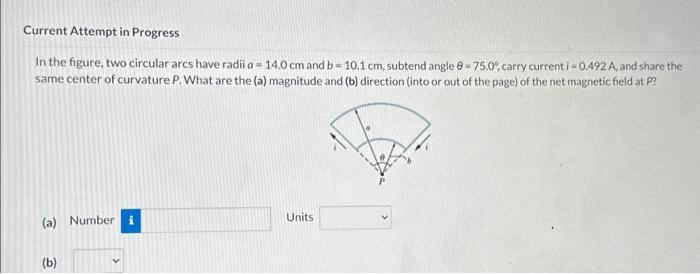# (Solved): In the figure, two circular arcs have radii a=14.0cm and b=10.1cm, subtend angle =75.0, ca ...In the figure, two circular arcs have radii and , subtend angle , carry current , and share the same center of curvature P. What are the (a) magnitude and (b) direction (into or out of the page) of the net magnetic field at P? (a) Number Units

We have an Answer from Expert